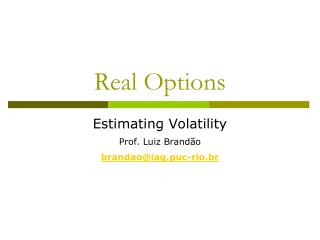# Real Options - PowerPoint PPT PresentationDownload PresentationReal Options

Real Options
Download Presentation## Real Options

- - - - - - - - - - - - - - - - - - - - - - - - - - - E N D - - - - - - - - - - - - - - - - - - - - - - - - - - -
##### Presentation Transcript

1. Real Options Estimating Volatility Prof. Luiz Brandão brandao@iag.puc-rio.br

2. Estimating Volatility

3. Example: InterSom Lda. • InterSom plans to launch a new line of products that will have an expected life of four years. • Expected cash flows in spreadsheet. • WACC is 10% and risk free rate is 5%. • The project has an option to expand in year 2 and can be abandoned for a fixed amount in years 2 and 3. • Sales are expected to grow 8% annually. • What is the value of the project considering the options?

4. Step 1: DCF Model

5. Step 2: Model Uncertainties • In this case the only uncertainty is the future level of sales. • We assume that sales revenues R follow a GBM. • The growth rate is 8.0% • We also assume that the volatility of revenues is σR = 30%. The model will then be: .xls

6. Step 3: Simulation • We model the returns v as: where • Run a simulation on the project returns • The project volatility is the standard deviation of the returns.

7. Step 4: Model the Project and Options • PV = 1178 • WACC = 10% • Volatility = ? • Assume the project has an option to expand 30% in year 2 at a cost of \$100, and can be abandoned for \$350

8. DPL Model • Underlying Asset Model is:

9. DPL Model with Options

10. Adding more sources of Uncertainty

11. DiaGenesis Ltd. • One of the advantages of the Monte Carlo simulation is that one can use more than one source of uncertainty. • These uncertainties can be of any nature, independent or correlated. • We will illustrate this through a practical model. • Consider the firm DiaGenesis that has the oportunity to invest \$20.000 in a five year project. • The WACC is 15% a.a. and the risk free rate is 5%. • The DCF analysis indicates that the Expected Present Value of the project is 20.056. • The project has an optionn to expand in year 2 and can be abandoned in years 2 and 3.

12. 0 1 2 3 4 5 Sales 8.000 8.841 9.771 10.799 11.935 13.190 Costs (2.000) (2.167) (2.347) (2.542) (2.754) (2.984) Oper Expenses (800) (800) (800) (800) (800) Depreciation (4.000) (4.000) (4.000) (4.000) (4.000) EBIT 1.875 2.624 3.456 4.380 5.406 Tax 40% (750) (1.050) (1.383) (1.752) (2.162) Net Income 1.125 1.575 2.074 2.628 3.244 Depreciation 4.000 4.000 4.000 4.000 4.000 CAPEX (20.000) Free Cash Flow (20.000) 5.125 5.575 6.074 6.628 7.244 V = 20.056 WACC = 15% 0 Setp 1 – Base Case

13. Two Sources of Uncertainty • Assume this project has two independent sources of uncertainty • Sales (S) • Stochastic Process: Geometric Brownian Motion • Growth (Drift) rate = 10% a.a. • Volatility = 40%. • Costs (C) • Stochastic Process: Geometric Brownian Motion • Growth (Drift) rate = 10% a.a. • Volatility =20%.

14. Sales Uncertainty • The MGB process for Sales is: • The Simulation Model is: where: • St = Sales in the previous year • μS= average growth rate • σS= Volatility

15. Cost Uncertainty • The MGB process for the Costs is: • The Simulation Model is: • Where: • Ct= Costs in the previous year • μS= average growth rate • σC= Volatility

16. Step 2 – Simulation Parameters • Sales: • Costs:

17. Steps 3 and 4 – Simulation and Modeling • The BDH simulation provides a volatility of 43.89% • For the binomial model in DPL, we must determine the dividend rate for each of the five years of the project. • The remaining parameters are: • V0 = 20.056 • r = 5% • Volatility = 43,89% • Dividend rate for each year. • Once the underlying project is modeled, we proceed to add the project options

18. Model of the Underlying Project

19. Adding the Options • The project can be expanded by 30% at the end of year 2 at a cost of \$5.000. • The project can be abandoned at the end of years 2 and 3, by receiveing a residual value of \$8,000. • With these options, the value of the project increases from \$20.056 to \$22.663,42.

20. Model with Options